Math 121 - Calculus for Biology I Fall Semester, 2003 Linear Models© 2001, All Rights Reserved, SDSU & Joseph M. Mahaffy San Diego State University -- This page last updated 07-Aug-02Linear Models

Outline of Chapter

Chirping Crickets and Temperature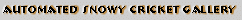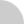....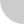.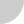For many years people have recognized a relationship between the temperature and the rate at which crickets are chirping. The folk method of determining the temperature in degrees Fahrenheit is to count the number of chirps in a minute and divide by 4, then add 40. In 1898, A. E. Dolbear  noted that "crickets in a field [chirp] synchronously, keeping time as if led by the wand of a conductor." In his paper, he appears to be the first person to write down a formula in a scientific publication, giving a linear relationship for the temperature based on the chirp rate of crickets. The mathematical formula that he gave is: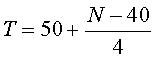Does this formula of Dolbear match the folk method described above?

Many of the early papers [1,2] begin with the authors' fond memories of listening to snowy tree crickets, Oecanthulus niveus, in the late summer and early fall, then they dispute how synchronized the actual chirping is. However, the mathematical models are all very similar.

Below is the data from C. A. Bessey and E. A. Bessey on eight different crickets that they observed in Lincoln, Nebraska during August and September, 1897. It is apparent from these data that a fairly good estimate of the temperature is found by drawing a straight line through the points. A Java applet is provided so that you can adjust the coefficients of the straight line. Change the coefficients of the equation of the line below until you see a line that looks to you as if it fits the data. This line would be a good model for finding the temperature outside based on the rate at which the crickets are chirping.

source for linear_ckt.java

The line given by the Bessey brothers is the least squares best fit to the data they collected. (The actual formula that they presented is T = 60 + (N - 92)/4.7, which you can check reduces to the formula stated in the graph above.) We will examine what a least squares best fit means in the next section.

Dolbear's Cricket Equation as a Linear Model

The line that you found passing through the data creates a mathematical model for representing the temperature as a function of the rate at which snowy tree crickets chirp. Before studying this model for mathematical properties, we should ask a few questions about the biological model.

1. How well does the line that you found fitting the Bessey & Bessey data agree with the Dolbear model given above?
2. When can this model be applied from a practical perspective?
3. Over what range of temperatures is this model valid?
4. How accurate is the model and how might the accuracy be improved?

The answers to these questions should help you appreciate the complex relationship between the biology of the problem and the mathematical model. (Check the Modeling diagram in the introduction.) The answers that are given below are not complete, but should help you appreciate how one approaches mathematical modeling and a biological problem. Hopefully, this will give you a better appreciation of how mathematics is used and some of its limitations.

The first two questions are actually very biological in nature, and the mathematics play a very limited role. The comparison of the Dolbear formula to the linear model shows some discrepancies in the coefficients of the linear model. However, you should be asking the biological question about the organisms that were being studied. The differences in the mathematical formulae may very well be due to observations made on different species of crickets. However, if you believe that the two different observations are similar, then this model may be a good biological thermometer. From a practical perspective, this biological thermometer has limited use. The snowy tree crickets only chirp for a couple months of the year. Furthermore, they only tend to chirp at night when the temperature is above 50oF.

The last two questions provide important links between the process of mathematical modeling and the biological problem being studied. The range of validity in temperatures for the model gives the domain where we can use this model. Generally, you should limit the use of the mathematical model to points between the range where the data are collected (or possibly to intervals that are only slightly beyond the collected data points). For our cricket thermometer equation, we see that the data only allows its use between 50oF and 85oF. However, this temperature range is appropriate for evenings in Nebraska in August and September, which is where this particular thermometer is valid. Statistical analysis of the data improves the degree of accuracy of the mathematical model, but it appears that the folk model will probably give the temperature within a couple degrees Fahrenheit. The folk formula is less accurate than the model formed by data, but it is much more easily applied. So which technique are you more likely to use on a warm summer night talking to some friends?

You created a model above by adjusting the slope and intercept of a linear equation until you saw a model that looks good. Mathematically, we often use a linear least squares fit to find the best fit to the data. The next chapter of these notes will show you how to obtain this best straight line through the data, using the technique of linear least squares and later chapters will discuss more complicated models. The data could be better fit by a more complicated mathematical model, such as fitting a quadratic through the data. However, this may not be appropriate from either a biological or mathematical perspective, but that depends on the problem and the ability to deal with this is acquired through experience. The next section below will provide a review of straight lines and remind you of some important mathematical definitions.

Equations of Lines

The general equation of a line is given by

y = mx + b.

This is commonly known as the slope-intercept form of the line. The variable x is known as the independent variable, and the variable y is known as the dependent variable. The slope is given by m, and the y-intercept is given by b. It is important to note that the variables x and y are only used for convenience. When describing a mathematical model using a linear model, one often chooses variables that more closely match the objects being observed.

The cricket equation given above can be writtenAs written, the independent variable is N, which is the rate that the crickets are chirping (number of chirps per minute). The temperature, T, is the dependent variable. The slope is 1/4, and the T-intercept is 40. A graph of this equation is seen in the applet above by pressing the button for Dolbear. This graph does not pass through the data, but it is not very far removed from the data. Thus, it can still be considered an appropriate model for estimating the temperature.

The point-slope form of a line is another common and useful form of the line. If a line passes through the point (x0, y0) and has a slope of m, then the equation of the line can be written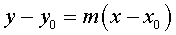From this form of the equation, it is easy to find the slope if you are given two points. Given the two points (x0 , y0) and (x1 , y1), then the slope m is given by:Examples reviewing lines can be found in the hyperlinked Worked Examples section. This section includes an applet to help you understand the connections between the graphs and the algebraic formula.

Metric System Conversion

All of the conversions for measurements, weights, temperatures, etc. are linear relationships. Most of the conversions only require a change in the slope as they agree at zero, but this is not the case for temperature. Below we use the information above on straight lines to determine a formula for finding the temperature in degrees Celsius as a function of the temperature in degrees Fahrenheit.

The United States is one of the few countries in the world that uses the Fahrenheit scale for temperature. The freezing point of water is 32oF and 0oC, so take (f0 , c0) = (32, 0). The boiling point of water is 212oF and 100oC (at sea level), so take (f1, c1) = (212,100). The slope is computed as follows: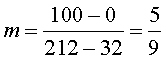Thus, the point-slope form of the line gives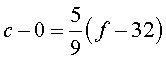orThe above formula takes any temperature f in Fahrenheit and converts to c in Celsius.

Below are JavaScript programs that you can use to find a number of transformations from one set of units to another. Underlying the code for all of these conversions is a linear relationship.

 Weight Volume Area Force Distance Temperature Pressure

Example: As an example of how you can use this applet to find a linear relationship, suppose that you want a formula to find the weight in pounds given the weight in kilograms.

Solution: By placing a 1 in the category of kilograms, you find that each kilogram is 2.2046 pounds. Thus, the linear relationship is simply 2.2046 times the weight in kilograms. If we let p be the weight in pounds and k be the weight in kilograms, the relationship is given by

p = 2.2046k.

Additional conversion problems can be found in the hyperlinked Worked Examples section.

Juvenile Height

In the table below we show average juvenile height as a function of age .

 Age 1 3 5 7 9 11 13 Height (cm) 75 92 108 121 130 142 155

The height, h, is graphed as a function of the age, a. The data from the table are shown below. It is easy to see that the data almost lie on a line, which suggests a linear model.

source for linear_hta.java

The Java applet allows you to adjust the coefficients of the linear model. Change the coefficients of the equation of the line above until the line, representing the height as a function of age, fits the data. This line would be a good model for finding the average height of a child for any age between one and thirteen.

The line that best fits the data above is given by

h = ma + b = 6.46a + 72.3,

where m = 6.46 is the slope and b = 72.3 is the h-intercept. The next section will explain finding the linear least squares best fit or linear regression to the data.

From a modeling perspective, it is often valuable to place units on each of the coefficients or variables in the equation. In an equation the units must always match. The height, h, from our data has units of cm, so both ma and the intercept b must have units cm. Since the age, a, has units of years, it follows that the slope, m, has units of cm/year. From the units it is easy to see that the slope is the rate of growth. (This idea of rate of growth will occur regularly in this course!)

The line above gives a mathematical model for growth of the average child. With this mathematical model, what type of questions can you answer? See if you can answer the following questions.

1. What is the average height of an eight year old?
2. What height does the model predict for a newborn baby?
3. If a six year old child is 110cm, then estimate how tall she'll be at age 7.

1. The model predicts that the average eight year old will be 124 cm, which is found by setting a = 8 in the model.

2. The height intercept represents the height of a newborn, so this model predicts that a newborn would be 72.3 cm. However, this is outside the range of the data, which makes its value more suspect.

3. The model indicates that the growth rate is about 6.5 cm/year, so the six year old should grow about 6.5 cm and be 116.5 cm at age 7 though the average 7 year old as predicted by the model would be 117.5 cm.

What are some of the limitations and how might the model be improved?

The most obvious limitation is that this linear model would certainly not extend much beyond the ages listed in the table. (You would not predict the average 20 year old to be 201.5 cm as the model predicts.) Thus, the domain of this function is restricted to some interval around 1 < a < 13.

Let's examine the questions above to see if we might derive better estimates. A better prediction of average eight year olds would be to average the heights of seven and nine year olds (125.5 cm). This is known as a local analysis, meaning that approximating a function is always better by using nearby information. Similarly, we might improve our estimate on the length of a newborn by using only the data given for one and three year olds (66.5 cm). As we study Calculus more, we will see that its this local study of growth rates that is of greatest interest. The answer to the third question is about as good as we can do with the given information. If you had more data on the individual child, you might be able to predict her height better from her history than using the history of this average set of children.

There are several improvements you might want in a model like this. (Recall that models are only a window on the real world and usually can be improved.) The model is an average of juveniles indicating that the data have both sexes included, and our experience suggests that growth rates for girls and boys differ. Thus, you might want to split the data according to sex. Close inspection of the data shows that there is a faster growth rate between 0 and 5, and then again between 9 and 13, which agrees with the common idea that growth occurs in spurts. You might improve the model to include this information by using something other than a straight line to fit the data. However, you must consider how much is gained by a more complicated model.

References

 H. A. Allard, The chirping rates of the snowy tree cricket (Oecanthus niveus) as affected by external conditions, Canadian Entomologist (1930) 52, 131-142.

 C. A. Bessey and E. A. Bessey, Further notes on thermometer crickets, American Naturalist (1898) 32, 263-264.

 A. E. Dolbear, The cricket as a thermometer, American Naturalist (1897) 31, 970-971.

 David N. Holvey, editor, The Merck Manual of Diagnosis and Therapy (1987) 15th ed., Merck Sharp & Dohme Research Laboratories, Rahway, NJ.

Top of Page